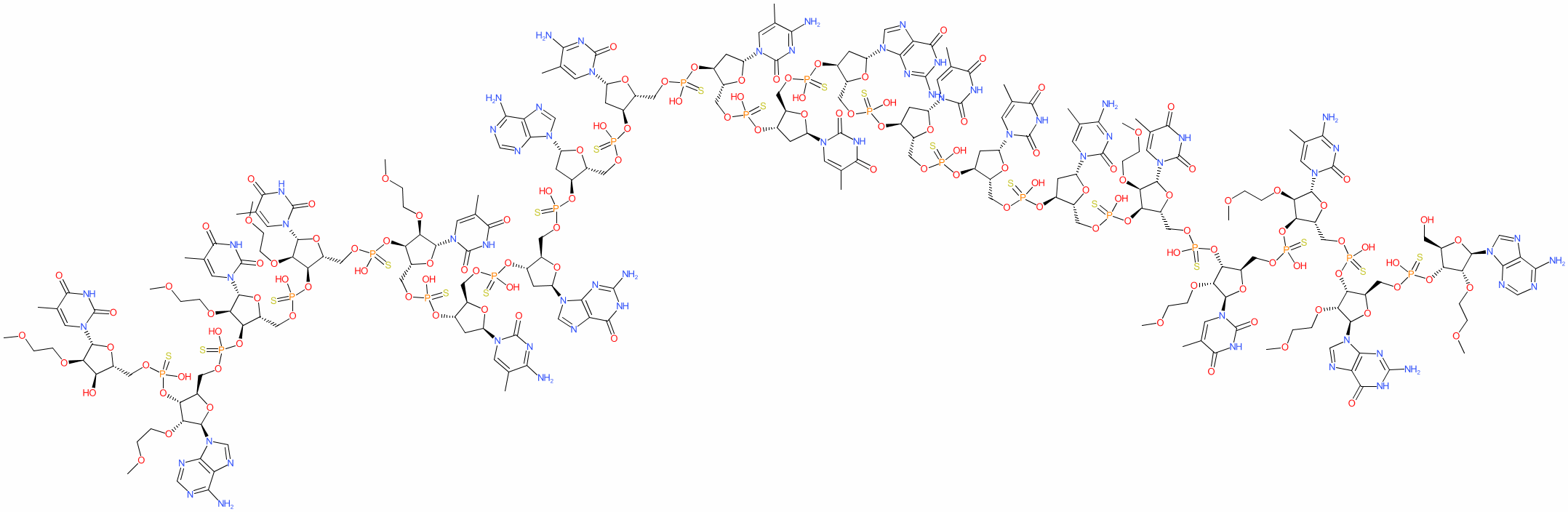#### GtoPdb Ligand ID: 8681

Synonyms: APOC-III antisense inhibitor | ISIS 304801 | ISIS-APOCIIIRx | Waylivra®
volanesorsen is an approved drug (EMA (2019))
Compound class: Synthetic organic
Comment: Volanesorsen is an antisense oligonucleotide (ASO) designed to reduce levels of apolipoprotein C-III (APOC3) in patients with elevated blood triglyceride levels . Reducing triglycerides via APOC3 could be beneficial in patients with inherited hypertriglyceridemia (e.g. those with familial chylomicronemia syndrome, FCS) . The role of APOC3 in hypertriglyceridemia and the effects APOC3 ASO use are reviewed in .
HELM annotation for this RNA (from PubChem) is RNA1{[MOE](A).[sp][MOE](G).[sp][MOE]([5meC]).[sp][MOE](T).[sp][MOE](T).[sp][dR]([5meC]).[sp][dR](T).[sp][dR](T).[sp][dR](G).[sp][dR](T).[sp][dR]([5meC]).[sp][dR]([5meC]).[sp][dR](A).[sp][dR](G).[sp][dR]([5meC]).[sp][MOE](T).[sp][MOE](T).[sp][MOE](T).[sp][MOE](A).[sp][MOE](T)}\$\$\$\$.
2D StructurePhysico-chemical PropertiesHydrogen bond acceptors 130 Hydrogen bond donors 44 Rotatable bonds 156 Topological polar surface area 3216.79 Molecular weight 7160.03 XLogP 6.58 No. Lipinski's rules broken 4
SMILES / InChI / InChIKeyCanonical SMILES COCCO[C@@H]1[C@H](OP(=S)(OC[C@H]2O[C@H](C[C@@H]2OP(=S)(OC[C@H]2O[C@H](C[C@@H]2OP(=S)(OC[C@H]2O[C@H](C[C@@H]2OP(=S)(OC[C@H]2O[C@H](C[C@@H]2OP(=S)(OC[C@H]2O[C@H](C[C@@H]2OP(=S)(OC[C@H]2O[C@H](C[C@@H]2OP(=S)(OC[C@H]2O[C@H](C[C@@H]2OP(=S)(OC[C@H]2O[C@H](C[C@@H]2OP(=S)(OC[C@H]2O[C@H](C[C@@H]2OP(=S)(OC[C@H]2O[C@H](C[C@@H]2OP(=S)(OC[C@H]2O[C@H]([C@@H]([C@@H]2OP(=S)(OC[C@H]2O[C@H]([C@@H]([C@@H]2OP(=S)(OC[C@H]2O[C@H]([C@@H]([C@@H]2OP(=S)(OC[C@H]2O[C@H]([C@@H]([C@@H]2OP(=S)(OC[C@H]2O[C@H]([C@@H]([C@@H]2O)OCCOC)n2cc(C)c(=O)[nH]c2=O)O)OCCOC)n2cnc3c2ncnc3N)O)OCCOC)n2cc(C)c(=O)[nH]c2=O)O)OCCOC)n2cc(C)c(=O)[nH]c2=O)O)OCCOC)n2cc(C)c(=O)[nH]c2=O)O)n2cc(C)c(nc2=O)N)O)n2cnc3c2nc(N)[nH]c3=O)O)n2cnc3c2ncnc3N)O)n2cc(C)c(nc2=O)N)O)n2cc(C)c(nc2=O)N)O)n2cc(C)c(=O)[nH]c2=O)O)n2cnc3c2nc(N)[nH]c3=O)O)n2cc(C)c(=O)[nH]c2=O)O)n2cc(C)c(=O)[nH]c2=O)O)n2cc(C)c(nc2=O)N)O)[C@H](O[C@H]1n1cc(C)c(=O)[nH]c1=O)COP(=S)(O[C@@H]1[C@@H](COP(=S)(O[C@@H]2[C@@H](COP(=S)(O[C@@H]3[C@@H](COP(=S)(O[C@@H]4[C@@H](CO)O[C@H]([C@@H]4OCCOC)n4cnc5c4ncnc5N)O)O[C@H]([C@@H]3OCCOC)n3cnc4c3nc(N)[nH]c4=O)O)O[C@H]([C@@H]2OCCOC)n2cc(C)c(nc2=O)N)O)O[C@H]([C@@H]1OCCOC)n1cc(C)c(=O)[nH]c1=O)O Isomeric SMILES COCCO[C@@H]1[C@H](OP(=S)(OC[C@H]2O[C@H](C[C@@H]2OP(=S)(OC[C@H]2O[C@H](C[C@@H]2OP(=S)(OC[C@H]2O[C@H](C[C@@H]2OP(=S)(OC[C@H]2O[C@H](C[C@@H]2OP(=S)(OC[C@H]2O[C@H](C[C@@H]2OP(=S)(OC[C@H]2O[C@H](C[C@@H]2OP(=S)(OC[C@H]2O[C@H](C[C@@H]2OP(=S)(OC[C@H]2O[C@H](C[C@@H]2OP(=S)(OC[C@H]2O[C@H](C[C@@H]2OP(=S)(OC[C@H]2O[C@H](C[C@@H]2OP(=S)(OC[C@H]2O[C@H]([C@@H]([C@@H]2OP(=S)(OC[C@H]2O[C@H]([C@@H]([C@@H]2OP(=S)(OC[C@H]2O[C@H]([C@@H]([C@@H]2OP(=S)(OC[C@H]2O[C@H]([C@@H]([C@@H]2OP(=S)(OC[C@H]2O[C@H]([C@@H]([C@@H]2O)OCCOC)n2cc(C)c(=O)[nH]c2=O)O)OCCOC)n2cnc3c2ncnc3N)O)OCCOC)n2cc(C)c(=O)[nH]c2=O)O)OCCOC)n2cc(C)c(=O)[nH]c2=O)O)OCCOC)n2cc(C)c(=O)[nH]c2=O)O)n2cc(C)c(nc2=O)N)O)n2cnc3c2nc(N)[nH]c3=O)O)n2cnc3c2ncnc3N)O)n2cc(C)c(nc2=O)N)O)n2cc(C)c(nc2=O)N)O)n2cc(C)c(=O)[nH]c2=O)O)n2cnc3c2nc(N)[nH]c3=O)O)n2cc(C)c(=O)[nH]c2=O)O)n2cc(C)c(=O)[nH]c2=O)O)n2cc(C)c(nc2=O)N)O)[C@H](O[C@H]1n1cc(C)c(=O)[nH]c1=O)COP(=S)(O[C@@H]1[C@@H](COP(=S)(O[C@@H]2[C@@H](COP(=S)(O[C@@H]3[C@@H](COP(=S)(O[C@@H]4[C@@H](CO)O[C@H]([C@@H]4OCCOC)n4cnc5c4ncnc5N)O)O[C@H]([C@@H]3OCCOC)n3cnc4c3nc(N)[nH]c4=O)O)O[C@H]([C@@H]2OCCOC)n2cc(C)c(nc2=O)N)O)O[C@H]([C@@H]1OCCOC)n1cc(C)c(=O)[nH]c1=O)O InChI Key IJUQCWMZCMFFJP-GQSLRNSLSA-N
 No information available.
 Summary of Clinical UsePhase 3 clinical trials NCT02300233 and NCT02211209 are assessing volanesorsen in patients with elevated triglyceride levels and with FCS respectively. Both the US FDA and EMA granted volanesorsen orphan designation for the treatment of FCS. Full EMA marketing authorisation as a treatment for hyperlipoproteinemia type I was granted in 2019 .